## 1 Introduction

Let $$\mathbb {N}= \{0,1,2,\ldots \}$$ denote the natural numbers and $$\mathbb {N}^+= \{1,2,3,\ldots \}$$ denote the positive integers. We define the Collatz function $$C :\mathbb {N}^+\rightarrow \mathbb {N}^+$$ as

\begin{aligned} C(n) = {\left\{ \begin{array}{ll} n/2 &{} \text {if } n \equiv 0 \pmod 2 \\ 3n + 1 &{} \text {if } n \equiv 1 \pmod 2. \end{array}\right. } \end{aligned}

Given a function f and a number $$k \in \mathbb {N}$$, the function $$f^k$$ denotes the kth iterate of f. The well-known Collatz conjecture is the following:

### Conjecture 1

For all $$n \in \mathbb {N}^+$$, there exists some $$k \in \mathbb {N}$$ such that $$C^k(n) = 1$$.

This is a longstanding open problem and there is a vast literature dedicated to its study. For its history, we refer the reader to the comprehensive surveys by Lagarias [17,18,19].

### Definition 1 (Convergent function)

Consider a function $$f :X \rightarrow X$$. Given $$x \in X$$, the sequence of iterates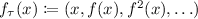is called the f-trajectory of x. For some designated element $$z \in X$$, if for all $$x \in X$$ the trajectory $$f_\tau (x)$$ contains z, the function f is called convergent.

In this paper, we describe an approach based on termination of string rewriting to automatically search for a proof of the Collatz conjecture. Although trying to prove the Collatz conjecture via automated deduction is clearly a moonshot goal, there are two recent technological advances that provide reasons for optimism that at least some interesting variants of the problem might be solvable. First, the invention of the method of matrix interpretations and its variants such as arctic interpretations turns the quest of finding a ranking function to witness termination into a problem that is suitable for systematic search. Second, the progress in satisfiability (SAT) solving makes it possible to solve many seemingly difficult combinatorial problems efficiently in practice. Their combination, i.e., using SAT solvers to find interpretations, has so far been effective in solving challenging termination problems. We make the following contributions:

• We show how a generalized Collatz function can be expressed as a rewriting system that is terminating if and only if the function is convergent.

• We show that translations into rewriting systems that use non-unary representations of numbers are empirically more amenable to automation compared with their previously and more commonly studied counterparts that use unary representations.

• We automatically prove various weakenings of the Collatz conjecture and observe that only relatively large matrix/arctic interpretations exist for some generalized Collatz functions. Existing termination tools often limit their default strategies to search for small interpretations as they are tailored for the setting where the task is to quickly solve a large quantity of relatively easy problems. We make the point that, given more resources, the interpretation method has the potential to scale.

• We observe that the phase-saving heuristic used in modern SAT solvers degrades the performance of CDCL solvers on formulas encoding the existence of matrix/arctic interpretations, whereas using negative branching improves solver performance.

• We present adaptations of our rewriting system that allow reformulating several more open problems in mathematics as termination problems of small size.

## 2 Preliminaries

### Definition 2 (String rewriting system)

Let $$\varSigma$$ be an alphabet, i.e., a set of symbols. A string rewriting system (SRS) over $$\varSigma$$ is a relation $$R \subseteq \varSigma ^* \times \varSigma ^*$$. Elements $$(\ell , r) \in R$$ are called rewrite rules and are usually written as $$\ell \rightarrow r$$. The system R induces a rewrite relation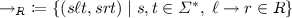on the set $$\varSigma ^*$$ of strings.

### Definition 3 (Termination)

A relation $$\mathord {\rightarrow }$$ on A is terminating (denoted $$\mathrm {SN}(\mathord {\rightarrow })$$) if there is no infinite sequence $$s_0, s_1, \ldots \in A$$ such that $$s_i \rightarrow s_{i+1}$$ for all $$i \ge 0$$.

We conflate an SRS R with the rewrite relation it induces, writing “R is terminating” instead of “$$\mathord {\rightarrow }_R$$ is terminating”. The following is a useful generalization of termination:

### Definition 4 (Relative termination)

For SRSs R and S, the system R is said to be terminating relative to S (denoted $$\mathrm {SN}(R/S)$$) if every sequence of rewrites for the system $$R \cup S$$ applies the rules from R at most finitely many times.

Relative termination allows proofs to be broken into steps as codified by the following.

### Lemma 1

(Rule removal [29, Theorem 1]). Let R be an SRS. If there exists a subset $$T \subseteq R$$ such that $$\mathrm {SN}(T/R)$$ and $$\mathrm {SN}(R \setminus T)$$, then $$\mathrm {SN}(R)$$.

This lemma allows us to “remove rules” in the following way. When proving $$\mathrm {SN}(R)$$, if we succeed at finding a subset T satisfying $$\mathrm {SN}(T/R)$$, the proof obligation becomes weakened to $$\mathrm {SN}(R \setminus T)$$, where the rules of T are no longer present. This removal of rules can be repeated until no rules remain, thus producing a stepwise proof of termination.

Another useful technique is reversal:

### Lemma 2

(Rule reversal [29, Lemma 2]). For a string $$s = s_1 \ldots s_n \in \varSigma ^*$$, denoteand define the reversal of an SRS R as. For SRSs R and S, we have $$\mathrm {SN}(R/S)$$ if and only if $$\mathrm {SN}({R}^\mathrm {rev}/{S}^\mathrm {rev})$$.

Reversal is of interest because methods for proving termination are not necessarily invariant under reversal, that is, a given technique may fail to show termination of a system R while succeeding for its reversal $${R}^\mathrm {rev}$$.

Yet another important notion is top termination:

### Definition 5 (Top termination)

Let R be an SRS over $$\varSigma$$. The top rewrite relation induced by R is defined as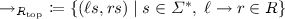. If $$\mathord {\rightarrow }_{{R}_\mathrm {top}}$$ is terminating, R is said to be top terminating.

In plain language, top termination allows rewrites to be performed only at the leftmost end of a string. As we will see in the next section (Theorem 1), top termination problems can admit proofs of a more relaxed form compared to termination. Relative top termination, i.e., proving $$\mathrm {SN}({R}_\mathrm {top}/S)$$ for SRSs R and S, is a crucial component in the dependency pair approach  which reduces a termination problem to a relative top termination problem that is often easier to solve. In order to avoid requiring familiarity with the dependency pair approach, we omit its discussion, and instead prove a self-contained result (Lemma 4) that encapsulates dependency pairs in a more elementary manner for the specific rewriting systems that we consider in this paper.

### 2.2 Interpretation Method

We state (at a high level) the key results on matrix/arctic interpretations that we use in our implementation. For more details we refer the reader to existing work [2, 6, 10, 15, 26]. With the interpretation method, the main idea is to find a ranking function that assigns a value to each string such that it decreases strictly when the string is modified by an application of a rewrite rule. If for all strings the value is bounded from below, then it cannot decrease indefinitely, ruling out the existence of an infinite sequence of rewrites. Formally, we search for an instance of the following:

### Definition 6 (Extended/weakly monotone algebra)

Let $$\varSigma$$ be an alphabet, A a set, $$[\sigma ] :A \rightarrow A$$ an interpretation for every $$\sigma \in \varSigma$$, > and $$\gtrsim$$ order relations over A such that > is well-founded and $$\gtrsim$$ satisfies $$\mathord {> \cdot \gtrsim } \subseteq \mathord {>}$$. Letting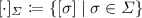, the structure $$(A, [\cdot ]_\varSigma , >, \gtrsim )$$ is a weakly monotone $$\varSigma$$-algebra if for every $$\sigma \in \varSigma$$ the interpretation $$[\sigma ]$$ is monotone with respect to $$\gtrsim$$. It is an extended monotone $$\varSigma$$-algebra if, additionally, for every $$\sigma \in \varSigma$$ the interpretation $$[\sigma ]$$ is monotone with respect to >.

We extend the interpretation from symbols to strings $$s = s_1 \ldots s_n \in \varSigma ^*$$ as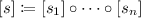. The following general theorem characterizes relative termination (resp. top termination) as the existence of extended (resp. weakly) monotone algebras.

### Theorem 1

([6, Theorem 2]). Let R and S be SRSs over the alphabet $$\varSigma$$. We have $$\mathrm {SN}(R/S)$$ (resp. $$\mathrm {SN}({R}_\mathrm {top}/S)$$) if and only if there exists an extended (resp. weakly) monotone $$\varSigma$$-algebra $$(A, [\cdot ]_\varSigma , >, \gtrsim )$$ such that

• for each rule $$\ell \rightarrow r \in R$$ we have $$[\ell ](x) > [r](x)$$ for all $$x \in A$$,

• for each rule $$\ell \rightarrow r \in S$$ we have $$[\ell ](x) \gtrsim [r](x)$$ for all $$x \in A$$.

An effective way to prove relative (top) termination is to try to satisfy the conditions of the above theorem by fixing $$(A, >, \gtrsim )$$ and algorithmically searching for appropriate interpretations of symbols. Matrix interpretations is an instance of this method. We fix a dimension d, set $$A = \mathbb {N}^d$$, define $$\vec {x}\gtrsim \vec {y}\iff x_i \ge y_i \text { for all } i \in \{1,\ldots ,d\}$$, and define $$\vec {x}> \vec {y}\iff \vec {x}\gtrsim \vec {y}\ \wedge \ x_1 > y_1$$. For interpreting each symbol $$\sigma \in \varSigma$$, we consider an affine function $$[\sigma ](\vec {x}) = M_\sigma \vec {x}+ v_\sigma$$. In this way, the structure ($$\mathbb {N}^d, [\cdot ]_\varSigma , >, \gtrsim$$) satisfies the requirements of Definition 6 for a weakly monotone algebra. Additionally setting $$(M_\sigma )_{1,1} = 1$$ satisfies the requirements for an extended monotone algebra. Matrix interpretations can also be adapted to the max–plus algebra of arctic numbersas coefficients with different arithmetic operations and order relations [15, 26].

### Example 1

Let $$R = \{aa \rightarrow aba\}$$ and $$S = \{b \rightarrow bb\}$$. The following functions constitute a matrix interpretations proof that shows $$\mathrm {SN}(R / S)$$.

\begin{aligned}{}[a](\vec {x}) = \begin{bmatrix} 1 &{} 1 \\ 0 &{} 0 \end{bmatrix} \vec {x}+ \begin{bmatrix} 0 \\ 1 \end{bmatrix} \qquad [b](\vec {x}) = \begin{bmatrix} 1 &{} 0 \\ 0 &{} 0 \end{bmatrix} \vec {x}+ \begin{bmatrix} 0 \\ 0 \end{bmatrix} \end{aligned}

It can be checked that the above interpretations give an extended monotone algebra and that they satisfy the following for all $$\vec {x}\in \mathbb {N}^2$$, which implies $$\mathrm {SN}(R/S)$$ via Theorem 1.

\begin{aligned}{}[aa](\vec {x}) = \begin{bmatrix} 1 &{} 1 \\ 0 &{} 0 \end{bmatrix} \vec {x}+ \begin{bmatrix} 1 \\ 1 \end{bmatrix} > \begin{bmatrix} 1 &{} 1 \\ 0 &{} 0 \end{bmatrix} \vec {x}+ \begin{bmatrix} 0 \\ 1 \end{bmatrix} = [aba](\vec {x}) \\ [b](\vec {x}) = \begin{bmatrix} 1 &{} 0 \\ 0 &{} 0 \end{bmatrix} \vec {x}+ \begin{bmatrix} 0 \\ 0 \end{bmatrix} \gtrsim \begin{bmatrix} 1 &{} 0 \\ 0 &{} 0 \end{bmatrix} \vec {x}+ \begin{bmatrix} 0 \\ 0 \end{bmatrix} = [bb](\vec {x}) \end{aligned}

In order to automate the search for the interpretations given a rewriting system R, an effective approach is to encode all of the aforementioned constraints as a propositional formula in CNF and use a SAT solver to look for a satisfying assignment. This additionally involves fixing a finite domain for the coefficients that can occur in the interpretations and encoding arithmetic over the chosen finite domain using propositional variables.

### 2.3 Generalized Collatz Functions

We consider instances of the following generalization of the Collatz function. Its variants have commonly appeared in the literature [3, 12, 14, 16, 21, 24, 27].

### Definition 7 (Generalized Collatz function)

Let X be one of $$\mathbb {N}$$, $$\mathbb {N}^+$$, or $$\mathbb {Z}$$ and define. A function $$f :X_\bot \rightarrow X_\bot$$ is a generalized Collatz function if $$f(\bot ) = \bot$$ and there exist an integer $$d \ge 2$$ and rational numbers $$q_0, \ldots , q_{d-1}, r_0, \ldots , r_{d-1}$$ such that for all $$0 \le i \le d-1$$ and all $$n \in X$$, we have

\begin{aligned} \begin{aligned}&f(n) = q_i n + r_i&\text {if } n \equiv i \pmod d \\ \text {or} \quad&f(n) = \bot&\text {if } n \equiv i \pmod d. \end{aligned} \end{aligned}

In the above, we allow the representation of a partially defined function by mapping to $$\bot$$ in the undefined cases. We call a partial f convergent if all f-trajectories contain $$\bot$$.

Note that the Collatz function corresponds to a generalized one with $$d = 2$$, $$q_0 = 1/2$$, $$r_0 = 0$$, $$q_1 = 3$$, $$r_1 = 1$$. Although the Collatz function is by far the most widely studied case, there are several other concrete examples of generalized Collatz functions the convergence of which is worth studying due to their connections to open problems in number theory and computability theory. We discuss these cases in Section 5.

## 3 Rewriting the Collatz Function

We start with systems that use unary representations and then demonstrate via examples that mixed base representations can be more suitable for use with automated methods.

### 3.1 Rewriting in Unary

The following system of Zantema  simulates iterated application of the Collatz function to a number represented in unary, and terminates upon reaching 1.

### Example 2

$$\mathcal {Z}$$ denotes the following SRS, consisting of 5 symbols and 7 rules.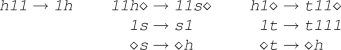This system can be seen as encoding the execution of a Turing machine with cells that can be contracted/expanded. The symbols 1 and(blank) form the tape alphabet, while the symbols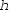(half),(shift),(triple) indicate the head along with the state of the machine. Through the following result, the Collatz conjecture can be reformulated as termination of string rewriting.

### Theorem 2

(). $$\mathcal {Z}$$ is terminating if and only if the Collatz conjecture holds.

While the forward direction of the above theorem is easy to see (since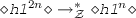for $$n > 1$$ andfor $$n \ge 0$$), the backward direction is far from obvious because not every string corresponds to a valid configuration of the underlying machine.

As another example, consider the system(originally due to ZantemaFootnote 1). Termination of this system has yet to be proved via automated methods. Nevertheless, there is a simple reason for its termination: It simulates iterated application of a partial generalized Collatz function $$W :\mathbb {N}^+_\bot \rightarrow \mathbb {N}^+_\bot$$ defined as follows, which is easily seen to be convergent.

\begin{aligned} W(n) = {\left\{ \begin{array}{ll} 3n/2 &{} \text {if } n \equiv 0 \pmod 2 \\ \bot &{} \text {if } n \equiv 1 \pmod 2 \end{array}\right. } \end{aligned}

If a proof of the Collatz conjecture is to be produced by some automated method that relies on rewriting, then that method better be able to prove a statement as simple as the convergence of W. With this in mind, we describe an alternative rewriting system that simulates the Collatz function and terminates upon reaching 1. We then provide examples where the alternative system is more suitable for use with termination tools (for instance allowing an automated proof of the convergence of W).

### 3.2 Rewriting in Mixed Base

In the mixed base scheme, the overall idea is as follows. Given a number $$n \in \mathbb {N}^+$$, we write a mixed binary–ternary representation for it (noting that this representation is not unique). With this representation, as long as the least significant digit is binary, the parity of the number can be recognized by checking only this digit, as opposed to scanning the entire string when working in unary. This allows us to easily determine the correct case when applying the Collatz function. If the least significant digit is ternary, then the representation is rewritten (while preserving its decimal value) to make this digit binary. Afterwards, since computing n/2 corresponds to erasing a trailing binary 0 and computing $$3n + 1$$ corresponds to inserting a trailing ternary 1, applying the Collatz function takes a single rewrite step. We explain this scheme more formally below.

A mixed base numeral system is a numeral system where the base changes across positions, which we define as follows. Note that unary is not a positional numeral system, so we require the bases to be greater than 1.

### Definition 8 (Mixed base representation)

Let $$B \subseteq \mathbb {N}_{>1}$$ be a set of bases and let $$N = {n_1}_{b_1} {n_2}_{b_2} \ldots {n_k}_{b_k}$$ be a string where $$n_i \in \mathbb {N}$$. If we have for each $$1 \le i \le k$$ that $$b_i \in B$$ and $$0 \le n_i < b_i$$, then N is called a mixed B-ary representation.

The string N from above represents the decimal number $$N_{10} = \sum _{i=1}^k n_i \prod _{j=i+1}^{k} b_j$$. Observing that the addition of leading zeros to a string does not change its decimal value, we may assume without loss of generality that $$n_1 > 0$$. Furthermore, $$b_1$$ does not affect the decimal value of the string, so we may omit it.

Now, define. After rearranging, we see that the decimal value of the B-ary string $$N = n_1 {n_2}_{b_2} \ldots {n_k}_{b_k}$$ may also be written as $$N_{10} = (\beta _{b_k}^{n_k} \circ \beta _{b_{k-1}}^{n_{k-1}} \circ \cdots \circ \beta _{b_2}^{n_2}) (n_1)$$. This gives us a string and a function view of the same representation, and we will switch between them as appropriate. In doing so, we also conflate the symbols and the corresponding functions, referring to $$\beta _b^n$$ as $$n_b$$.

As the last ingredient before describing the rewriting system, we observe that we can write $$(\beta _b^{n} \circ \beta _c^{m})(x) = bcx+bm+n$$ equivalently as another composition $$(\beta _c^{m'} \circ \beta _b^{n'})(x) = cbx+cn'+m'$$ for some suitable $$0 \le n' < b$$ and $$0 \le m' < c$$. This allows us to swap the bases of adjacent positions while preserving the decimal value of the string.

From this point on, we constrain ourselves to the mixed $$\{2,3\}$$-ary (binary–ternary) representations as we shift our focus to simulating the Collatz function (noting that it is possible to adapt the rewriting system that we will end up with to other instances of the general case). More precisely, we simulate the following redefinition of the Collatz function where the odd case incorporates an additional division by 2.

\begin{aligned} T(n) = {\left\{ \begin{array}{ll} \frac{n}{2} &{} \text {if } n \equiv 0 \pmod 2 \\ \frac{3n + 1}{2} &{} \text {if } n \equiv 1 \pmod 2 \end{array}\right. } \end{aligned}

We will describe an SRS $$\mathcal {T}$$ over the symbols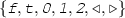that simulates iterated application of the Collatz function and terminates upon reaching 1. The symbols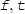correspond to binary digits $$0_2, 1_2$$; and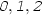to ternary digits $$0_3, 1_3, 2_3$$. The symbolmarks the beginning of a string while also standing for the most significant digit (without loss of generality assumed to be 1) and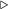marks the end of a string. Consider the functional view of these symbols:(1)

Each positive natural number can be expressed as some composition of these functions, which corresponds to a string as per our previous discussion.

### Example 3

Allowing the inclusion of a redundant trailing symbolto mixed base representations, we can write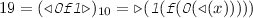. The string representation ends with a ternary symbol, so we will rewrite it. With the function view, we have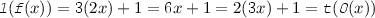. This shows that we could also write, which now ends with the binary digit $$1_2$$. This gives us the rewrite rule. We can now apply the Collatz function to this representation by rewriting only the rightmost two symbols of the string since. This gives us the rewrite rule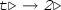. After applying this rule, we indeed obtain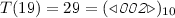.

In the manner of the above example, we compute all the necessary transformations and obtain the following 11-rule SRS $$\mathcal {T}$$.This SRS is split into subsystems $$\mathcal {D}_T$$ (dynamic rules for T) and $$\mathcal {X}= \mathcal {A}\cup \mathcal {B}$$ (auxiliary rules). The two rules in $$\mathcal {D}_T$$ encode the application of the Collatz function T, while the rules in $$\mathcal {X}$$ serve to push binary symbols towards the rightmost end of the string by swapping the bases of adjacent positions without changing the represented value.

### Example 4

(Rewrite sequence of $$\mathcal {T}$$). Consider the string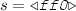that represents the number 12. Below is a possible rewrite sequence of $$\mathcal {T}$$ that starts from s, with the corresponding decimal values (under the interpretations from (1)) displayed above the strings. Underlines indicate the parts of the strings where the rules are applied.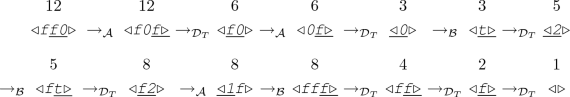The trajectory of T continues upon reaching 1, however, in order to be able to formulate the Collatz conjecture as a termination problem, $$\mathcal {T}$$ is made in such a way that its rewrite sequences stop upon reaching the string representation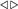of 1 since no rule is applicable.

Termination of the subsystems of $$\mathcal {T}$$ with $$\mathcal {B}$$ or $$\mathcal {D}_T$$ removed is easily seen. However, since we have matrix interpretations at our disposal, let us give a compact proof.

### Lemma 3

$$\mathrm {SN}(\mathcal {T}\setminus \mathcal {B})$$ and $$\mathrm {SN}(\mathcal {T}\setminus \mathcal {D}_T)$$.

### Proof

It is easily checked that the interpretations below show $$\mathrm {SN}({(\mathcal {T}\setminus \mathcal {B})}^\mathrm {rev})$$, which implies $$\mathrm {SN}(\mathcal {T}\setminus \mathcal {B})$$ by Lemma 2.Below interpretations show $$\mathrm {SN}({(\mathcal {T}\setminus \mathcal {D}_T)}^\mathrm {rev})$$, which implies $$\mathrm {SN}(\mathcal {T}\setminus \mathcal {D}_T)$$ by Lemma 2.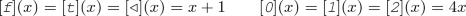$$\square$$

As a whole, the system $$\mathcal {T}$$ simulates the iterated application of T (except at 1).

### Theorem 3

$$\mathcal {T}$$ is terminating if and only if T is convergent.

### Proof (sketch)

We observe that the rules of $$\mathcal {T}$$ do not change the number of occurrences oforin a string and that the rewrite sequences operate strictly on one side of these symbols. Thus, we may view a given string as split into blocks delimited byorand consider the termination of each block separately. In this way, we conclude that there exists a nonterminating rewrite sequence for a string if and only if it contains a block of the canonical form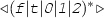that can be rewritten indefinitely, since the rewrite sequences that start on blocks of all other forms are already seen to terminate by Lemma 3. Furthermore, under the interpretations in (1), the sequences of values attained by the rewrites of the blocks in canonical form correspond directly to Collatz trajectories, since the rules in $$\mathcal {X}$$ do not change the value of the block and the rules in $$\mathcal {D}_T$$ change the value of the block in exactly the same way as the Collatz function T.    $$\square$$

When trying to remove a rule in $$\mathcal {D}_T$$ or $$\mathcal {B}$$ it suffices to show relative top termination, allowing us to use weakly (instead of extended) monotone algebras when applying Theorem 1 and take advantage of the more relaxed constraints when searching for matrix/arctic interpretations. The lemma below encapsulates dependency pairs, and it can in fact be automatically proved via the dependency pair framework .

### Lemma 4

For each subset $$\mathcal {R}\subseteq \mathcal {B}$$, if $$\mathrm {SN}(\mathcal {R}_\mathrm {top} / \mathcal {T})$$ then $$\mathrm {SN}(\mathcal {R}/ \mathcal {T})$$. And, for each subset $$\mathcal {R}\subseteq \mathcal {D}_T$$, if $$\mathrm {SN}({\mathcal {R}}^\mathrm {rev}_\mathrm {top} / {\mathcal {T}}^\mathrm {rev})$$ then $$\mathrm {SN}({\mathcal {R}}^\mathrm {rev} / {\mathcal {T}}^\mathrm {rev})$$.

### Proof (sketch)

Without loss of generality, assume we start with a string of the canonical form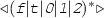(resp. its reversal). Then, the rules in $$\mathcal {B}$$ (resp. $${\mathcal {D}_T}^\mathrm {rev}$$) can only be applied at the top level. As we know from Lemma 3 that $$\mathcal {T}\setminus \mathcal {B}$$ (resp. $$\mathcal {T}\setminus \mathcal {D}_T$$) is terminating, any infinite sequence of rewrites in $$\mathcal {T}$$ (resp. its reversal) would require infinitely many applications of the rules from $$\mathcal {B}$$ (resp. $${\mathcal {D}_T}^\mathrm {rev}$$). As these rules can only be applied at the top level, this would imply relative top nontermination.    $$\square$$

## 4 Automated Proofs

We adapt the rewriting system $$\mathcal {T}$$ to different generalized Collatz functions to explore the effectiveness of the mixed base scheme on weakened variants of the Collatz conjecture. The rewriting systems, scripts to reproduce the experiments, and our implementation of a termination prover are available at https://github.com/emreyolcu/rewriting-collatz.

Most top-tier termination tools, such as AProVE, Matchbox, and, use the SAT solver MiniSat  to search for matrix/arctic interpretations. This choice is somewhat surprising as MiniSat has not been updated since 2008 and the performance of SAT solvers has improved significantly in the last decade. The use of MiniSat in these provers is motivated by its observed effectiveness in finding interpretations. We investigated the reason for this, which turned out to be a heuristic that MiniSat disables in its default configuration. MiniSat uses negative branching , which explores the “false” branch first for all decision variables. Modern SAT solvers use phase-saving  which first explores the branch corresponding to the truth value to which the variable was forced to most recently during unit propagation. In our case, enabling negative branching improves solver performance for formulas that encode the existence of interpretations.

### 4.1 Convergence of W

With the mixed binary–ternary scheme, the function W from Section 3.1 can be seen to be simulated by the system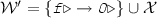. A small matrix interpretations proof is found for this system in less than a second, in contrast to its variant $$\mathcal {W}$$ that uses unary representations for which no automated proof is known.

### Theorem 4

$$\mathrm {SN}(\mathcal {W}')$$.

### Proof

The interpretations below prove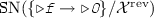: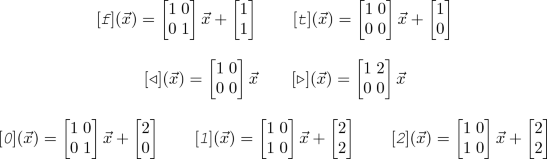By Lemmas 3 and 2, $${\mathcal {X}}^\mathrm {rev}$$ is terminating. As a result, $${\mathcal {W}'}^\mathrm {rev}$$ is terminating, which by Lemma 2 implies that $$\mathcal {W}'$$ is terminating.    $$\square$$

### 4.2 Farkas’ Variant

Let $${2\mathbb {N}+ 1}= \{1,3,5,\ldots \}$$ denote the odd natural numbers. Farkas  studied a slight modification $$F' :{2\mathbb {N}+ 1}\rightarrow {2\mathbb {N}+ 1}$$ of the Collatz function which can be proved convergent via induction. We consider automatically proving the convergence of this function as another test case for the mixed base scheme that is easier than the Collatz conjecture without being entirely trivial. We refer the reader to  for the original definition of $$F'$$. Below, we define another function $$F :\mathbb {N}\rightarrow \mathbb {N}$$ that resembles the Collatz function more closely than Farkas’ $$F'$$ (with respect to the definitions of the cases) while being equivalent to $$F'$$ in terms of convergence. This variant is obtained by introducing an additional case in the Collatz function for $$n \equiv 1 \pmod 3$$ and applying T otherwise. Its definition and a set $$\mathcal {D}_F$$ of dynamic rules are shown below.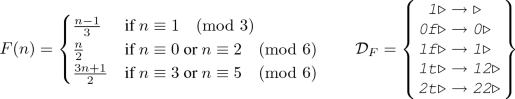Termination of the rewriting system $$\mathcal {F}= \mathcal {D}_F \cup \mathcal {X}$$ is equivalent to the convergence of F. The proof of the equivalence is essentially the same as that of Theorem 3. Farkas gave an inductive proof of convergence for $$F'$$ via case analysis, and we found an automated proof that $$\mathcal {F}$$ is terminating via arctic interpretations. It is worth mentioning that the default configurations of the existing termination tools (e.g., AProVE, Matchbox) are too conservative to prove termination of this system, but after their authors tweaked the strategies they were also able to find automated proofs via arctic interpretations.

### Theorem 5

For all $$n \in \mathbb {N}^+$$, the trajectory $$F_\tau (n)$$ contains 1.

### Proof

We will show $$\mathrm {SN}(\mathcal {F})$$. By Lemmas 3 and 2, we have $$\mathrm {SN}({\mathcal {X}}^\mathrm {rev})$$. The arctic interpretations below (with the empty cells standing for $$-\infty$$) prove $$\mathrm {SN}({\mathcal {D}_F}^\mathrm {rev}_\mathrm {top} / {\mathcal {X}}^\mathrm {rev})$$ by Theorem 1, which implies $$\mathrm {SN}({\mathcal {D}_F}^\mathrm {rev} / {\mathcal {X}}^\mathrm {rev})$$ by Lemma 4. As we know $${\mathcal {X}}^\mathrm {rev}$$ is terminating, by Lemma 1 we conclude $$\mathrm {SN}({\mathcal {D}_F}^\mathrm {rev} \cup {\mathcal {X}}^\mathrm {rev})$$, implying $$\mathrm {SN}(\mathcal {F})$$ via Lemma 2.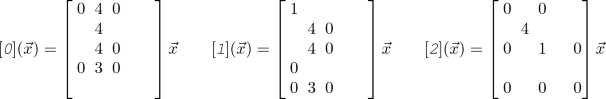### 4.3 Subsets of $$\mathcal {T}$$

It is also interesting to consider whether we can automatically prove terminations of proper subsets of $$\mathcal {T}$$. Specifically, we considered the 11 subsystems obtained by leaving out a single rewriting rule from $$\mathcal {T}$$, and we found proofs via matrix/arctic interpretations for all of the 11 subproblems. The reason for our interest in these problems is threefold:

1. 1.

Termination of $$\mathcal {T}$$ implies the terminations of all of its subsystems, so proving its termination is at least as difficult a task as proving terminations of the 11 subsystems. Therefore, the subproblems serve as additional sanity checks that an automated approach aspiring to succeed for the Collatz conjecture ought to be able to pass.

2. 2.

When proving termination in a stepwise manner, we solve a sequence of relative termination problems. Having proved the terminations of all 11 subsystems is a partial solution to the full problem, since it implies that for any single rule $$\ell \rightarrow r \in \mathcal {T}$$, proving $$\mathrm {SN}(\{\ell \rightarrow r\}/\mathcal {T})$$ settles the Collatz conjecture.

3. 3.

After the removal of a rule, the termination of the remaining system still encodes a valid mathematical question about the Collatz trajectories. The question of termination of a proper subset is equivalent to asking if every corresponding Collatz trajectory that does not require the use of the left-out rule is convergent.

### Example 5

As an instance of leaving out a rule, consider the subsystem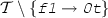. There is a single-step matrix interpretations proof that this system is terminating: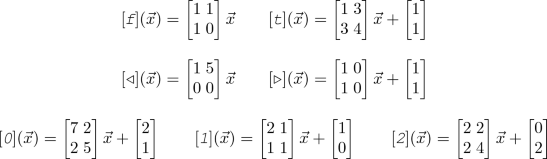With the above interpretations, we can show for instance that the Collatz trajectory starting at 3 (represented as) is convergent, because the missing rule is not used in any derivation of 1 () from 3. Below is an example derivation along with the decimal values each string represents and a vector value of each string under the interpretations above (setting $$\vec {x}= (0, 0)$$ for the purpose of demonstration). We omit the subscripts from the rewrite relations and simply write $$\mathord {\rightarrow }$$.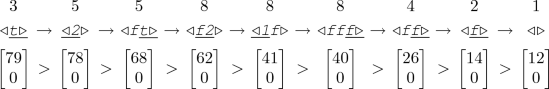Table 1 shows the parameters for the proofs that we found for the termination of each subsystem. For each rule $$\ell \rightarrow r$$ that is left out, we searched for a stepwise proof to show that $$\mathcal {B}\setminus \{\ell \rightarrow r\}$$ is terminating relative to $$\mathcal {T}\setminus \{\ell \rightarrow r\}$$ (freely utilizing weakly monotone algebras due to Lemma 4). Such a proof requires at most three steps since there are at most three rules in $$\mathcal {B}\setminus \{\ell \rightarrow r\}$$. On the table, we report the smallest parameters (in terms of matrix dimension) that work for all of these steps. As we already know that $$\mathrm {SN}(\mathcal {T}\setminus \mathcal {B})$$ holds (by Lemma 3), the interpretations found allow us to conclude the termination of each subsystem. This is not the only way to prove the terminations of the subsystems, however, we chose this uniform strategy for the sake of comparison.

### 4.4 Odd Trajectories

In the originally defined Collatz function C, applying $$2n+1 \mapsto 6n+4$$ produces an even number, so we incorporate a single division by 2 into the definition of the odd case and obtain the function T with the same overall dynamics as C. Taking this idea further by performing as many divisions by 2 as possible leads to the so-called Syracuse function $$\mathrm {Syr}:{2\mathbb {N}+ 1}\rightarrow {2\mathbb {N}+ 1}$$, defined as $$\mathrm {Syr}(n) = \frac{3n+1}{2^k}$$ where $$k = \max \{k \in \mathbb {N}^+\mid 2^k \text { divides } 3n+1\}$$.

Expressing the Syracuse function as a generalized Collatz function would require infinitely many cases to account for all of the possible appearances of $$2^k$$ as the denominator with different values of k. As a result, we are unable to simulate it with a finite rewriting system. Nevertheless, we may compromise and accelerate the Collatz function by a constant amount. We first observe that if $$n \equiv 1 \pmod 8$$ then $$\mathrm {Syr}(n) = \frac{3n+1}{4}$$ and if $$n \equiv 3 \pmod 4$$ then $$\mathrm {Syr}(n) = \frac{3n+1}{2}$$. Furthermore, for any $$n \in \mathbb {N}$$ we have $$\mathrm {Syr}(8n+5) = \mathrm {Syr}(2n+1)$$ since $$3(8n+5)+1 = 24n+16 = 4(6n+4) = 4(3(2n+1)+1)$$. Putting these observations together, we can define a generalized Collatz function $$S :{2\mathbb {N}+ 1}\rightarrow {2\mathbb {N}+ 1}$$ as follows.

\begin{aligned} S(n) = {\left\{ \begin{array}{ll} \frac{3n+1}{4} &{} \text {if } n \equiv 1 \pmod {8} \\ \frac{n-1}{4} &{} \text {if } n \equiv 5 \pmod {8} \\ \frac{3n+1}{2} &{} \text {if } n \equiv 3 \pmod {4} \end{array}\right. } \end{aligned}

S is convergent if and only if C (or T) is convergent, and the number of steps that S takes to converge is between that of T and $$\mathrm {Syr}$$. In a manner similar to before, we can translate S into a rewriting system. Since we are working with odd numbers we used a new symbolto mark the end of a string, viewed functionally as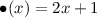. Termination of the rewriting system $$\mathcal {S}$$ is equivalent to the convergence of S. Similar to $$\mathcal {T}$$, proving the termination of $$\mathcal {S}$$ is currently beyond our reach, although it may potentially be an easier path to the Collatz conjecture (compared to proving $$\mathrm {SN}(\mathcal {T})$$). Failing to prove the termination of $$\mathcal {S}$$ itself, we considered the subsystems of $$\mathcal {S}$$ as we did for $$\mathcal {T}$$ in Section 4.3. With matrix/arctic interpretations, the terminations of all but two of the 11-rule subsystems of $$\mathcal {S}$$ were automatically proved. Despite devoting thousands of CPU hours, we were not able to find interpretations to prove that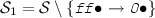oris terminating, so we leave them as challenges for automated termination proving.

### 4.5 Collatz Trajectories Modulo 8

Let m be a power of 2. Given $$k \in \{0, 1, \ldots , m-1\}$$, is it the case that all nonconvergent Collatz trajectories contain some $$n \equiv k \pmod m$$? For several values of k this can be proved to hold by inspecting the transitions of the iterates in the Collatz trajectories across residue classes modulo m (shown on Figure 1 for $$m = 8$$). These questions can also be formulated as the terminations of some rewriting systems. With this approach we found automated proofs for several cases:

### Theorem 6

If there exists a nonconvergent Collatz trajectory, it cannot avoid the residue classes of 2, 3, 4, 6 modulo 8.

It remains open whether the above holds for the residue classes of 0, 1, 5, 7 modulo 8.

## 5 More Problems to Approach via Rewriting

Mahler’s 3/2 Problem. Let $$\xi \in \mathbb {R}_{>0}$$ be a real number. It is called a Z-number if for all $$k \in \mathbb {N}$$ we have $$\mathrm {frac}\left( \xi \left( \frac{3}{2}\right) ^k \right) < \frac{1}{2}$$, where $$\mathrm {frac}(\cdot )$$ denotes the fractional part of the number. Mahler  conjectured that there are no Z-numbers. Moreover, he considered a generalized Collatz function $$M :\mathbb {N}^+ \rightarrow \mathbb {N}^+$$, defined as follows.

\begin{aligned} M(n) = {\left\{ \begin{array}{ll} \frac{3n}{2} &{} \text {if } n \equiv 0 \pmod 2 \\ \frac{3n+1}{2} &{} \text {if } n \equiv 1 \pmod 4 \\ \bot &{} \text {if } n \equiv 3 \pmod 4 \end{array}\right. } \end{aligned}

He related the behaviors of M-trajectories to the existence of Z-numbers:

### Theorem 7

For $$n \in \mathbb {N}^+$$, if a Z-number exists in the interval $$[n, n+1)$$, then there is no $$k \in \mathbb {N}$$ for which $$M^k(n) \equiv 3 \pmod 4$$.

Thus, the nonexistence of Z-numbers can be established by proving that M is convergent, which is equivalent to the termination of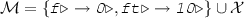. In order to ensure termination at the case $$n \equiv 3 \pmod 4$$, there is no rule with the LHS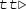.

Halting Problem for Busy Beaver-5. The busy beaver problem concerns finding binary-alphabet Turing machines with n states that, when given an input tape of all 0s, write the largest number of 1s on the tape upon halting. For each n, the machine that achieves this is called the “Busy Beaver-n”. Note that this definition only requires the machines to halt on all-0 inputs, leaving the behavior on other inputs unspecified and allowing them not to halt in general. Michel  observed that for $$n \in \{2, 3, 4\}$$, the busy beaver machines are all total Turing machines, i.e., they halt on all inputs, and moreover proved that they all simulate some generalized Collatz function. It is an open problem whether all busy beavers are total. In particular, it is unknown whether the current Busy Beaver-5 candidate is total. Michel showed that the Busy Beaver-5 candidate simulates the following generalized Collatz function.

\begin{aligned} B(n) = {\left\{ \begin{array}{ll} \frac{5n+18}{3} &{} \text {if } n \equiv 0 \pmod 3 \\ \frac{5n+22}{3} &{} \text {if } n \equiv 1 \pmod 3 \\ \bot &{} \text {if } n \equiv 2 \pmod 3 \end{array}\right. } \end{aligned}

Convergence of the above function can be studied via the termination of a rewriting system obtained by a mixed $$\{3,5\}$$-ary (ternary–quinary) translation scheme. We were unable to prove the termination of the resulting system.

Ternary Expansions of $$2^n$$. Erdős  asked: When does the ternary expansion of $$2^n$$ omit the digit 2? This is the case for $$2^0 = (1)_3$$, $$2^2 = (11)_3$$, and $$2^8 = (100111)_3$$. He conjectured that it does not happen for $$n > 8$$. This conjecture can be proved by showing that the rewriting systemis terminating on all initial strings of the form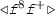. Given a string that corresponds to the binary representation of a power of 2, this system essentially rewrites the string into ternary by pushing ternary symbols to the right without altering the value that the string represents, and removes the occurrences of the ternary digits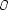and(but not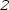). If the ternary expansion does not contain the digitthen all digits will be removed, resulting in the stringthat can then be rewritten to itself indefinitely. This problem, as described, is an instance of “local termination”  since it is concerned with termination on not all possible strings but a subset of them. We have not performed experiments with this system or local termination yet and we leave this for future work.

## 6 Related Work

To our knowledge, Zantema , with his system $$\mathcal {Z}$$ that we saw in Section 3.1, was the first to attempt using an automated method and string rewriting to search for a proof of the Collatz conjecture. In addition, although we independently discovered the mixed binary–ternary system described in Section 3.2, Scollo  had essentially the same idea, the difference being that he adopted a functional view of the digits that is slightly different than in (1). Scollo was not concerned with proving termination, though, and proposed rewriting primarily as a formalism that forgoes the arithmetic interpretation of the iterates and instead emphasizes its dynamic/computational behavior.

De Mol  showed the existence of a small 2-tag system  with the following rules that simulates the iterated application of the Collatz function given a unary representation: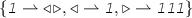. This tag system halts if and only if the Collatz conjecture holds, giving yet another formulation of the problem.

Kari  designed 1D cellular automata that perform multiplication by 3 and 3/2 in base 6, and reformulated both the Collatz conjecture and Mahler’s 3/2 problem as sets of constraints to be satisfied by the space-time diagrams of these cellular automata.

Kauffman  developed a formalism to perform arithmetic that he called string arithmetic, and expressed the Collatz conjecture within it. This formalism works with unary representations of numbers, and uses the three symbols,,. Lettingdenote the empty string and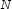be any string representing a number, string arithmetic consists of the following bidirectional rewrite rules (or “identities”) to convert between different strings representing the same number:. Then, the Collatz function is encoded by the following two rules: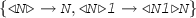. The Collatz conjecture is equivalent to the question of whether for strings ofs of all lengths there exists a rewrite sequence using the five rules above to reach the string.

## 7 Future Work

Several extensions to this work can further our understanding of the potential of rewriting techniques for answering mathematical questions. For instance, although matrix/arctic interpretations lead to automated proofs of several weakened variants discussed in this paper, it might still be the case that there exists no matrix/arctic interpretation to establish the termination of the Collatz system $$\mathcal {T}$$. Proving nonexistence would provide guidance as to where to focus our efforts when searching for a proof. Another issue is the matter of representation, specifically, it is worth exploring whether there exists a suitable translation of the Collatz conjecture into a term, instead of string, rewriting system since many automated termination proving techniques are generalized to term rewriting. Finally, injecting problem-specific knowledge into the rewriting systems or the termination techniques would be helpful as there exists a wealth of information about the Collatz conjecture that could simplify proof search.